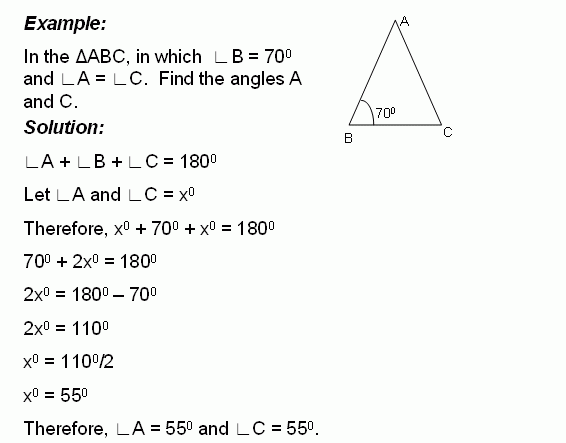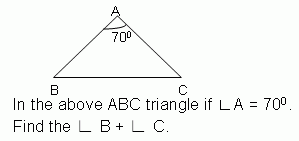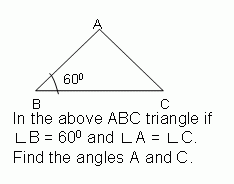Email us to get an instant 20% discount on highly effective K-12 Math & English kwizNET Programs!

#### Online Quiz (WorksheetABCD)

Questions Per Quiz = 2 4 6 8 10

### Middle/High School Algebra, Geometry, and Statistics (AGS)6.14 Find the Angles of TriangleDirections: Solve the following problems. Also write at least 10 examples of your own.
 Q 1:60 degrees70 degrees65 degrees Q 2:100 degrees105 degrees110 degrees Q 3:45 degrees60 degrees55 degrees Q 4: Find the angles of the triangle and classify based on the angles.45, 45, 9030, 60, 9020, 60, 100 Q 5:100 degrees90 degrees95 degrees Q 6: The measure of angle x is _____ degrees.Answer: Q 7: Find the angles of the triangle and classify based on the angles.32, 96, right angle triangle30, 60, right angle triangle26, 64, right angle triangle Q 8:75 degrees65 degrees60 degrees Question 9: This question is available to subscribers only! Question 10: This question is available to subscribers only!